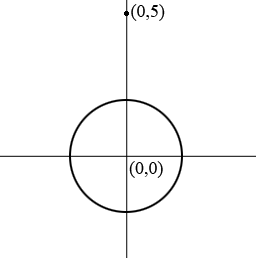SEARCH HOMEMath Central Quandaries & QueriesQuestion from mikee, a student: find the equation of a circle tangent to the circle x2+y2=4 and with the center at (0,5)Hi Mikee,

The circle $x^2 + y^2 = 4$

has center $(0,0)$ and radius $\sqrt{4} = 2.$ I drew a diagram.What is the radius of a circle with center $(0,5$ and tangent to the circle in the diagram? What is its equation.

Why does the problem say "find the equation of A circle..." rather than "find the equation of THE circle..."? Is there more than one answer?

PennyMath Central is supported by the University of Regina and the Imperial Oil Foundation.